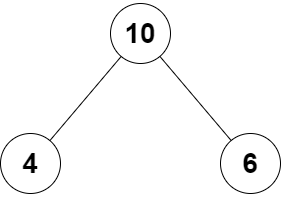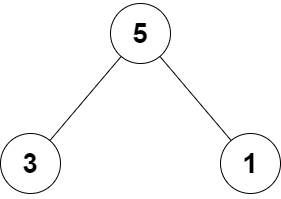##### Welcome to Subscribe On Youtube

Formatted question description: https://leetcode.ca/all/2236.html

# 2236. Root Equals Sum of Children (Easy)

You are given the root of a binary tree that consists of exactly 3 nodes: the root, its left child, and its right child.

Return true if the value of the root is equal to the sum of the values of its two children, or false otherwise.

Example 1:Input: root = [10,4,6]
Output: true
Explanation: The values of the root, its left child, and its right child are 10, 4, and 6, respectively.
10 is equal to 4 + 6, so we return true.


Example 2:Input: root = [5,3,1]
Output: false
Explanation: The values of the root, its left child, and its right child are 5, 3, and 1, respectively.
5 is not equal to 3 + 1, so we return false.


Constraints:

• The tree consists only of the root, its left child, and its right child.
• -100 <= Node.val <= 100

Related Topics:
Tree, Binary Tree

## Solution 1.

// OJ: https://leetcode.com/problems/root-equals-sum-of-children/
// Time: O(1)
// Space: O(1)
class Solution {
public:
bool checkTree(TreeNode* root) {
return root->val == root->left->val + root->right->val;
}
};